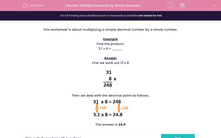# Multiply Decimals by Whole Numbers

In this worksheet, students multiply a simple decimal number by a whole number.Key stage:  KS 2

Curriculum topic:   Maths and Numerical Reasoning

Curriculum subtopic:   Decimals

Difficulty level:#### Worksheet Overview

This worksheet is about multiplying a simple decimal number by a whole number.

Example

Find the product.

3.1 x 8 = ____

First we work out 31 x 8Then we deal with the decimal point as follows.The answer is 24.8

### What is EdPlace?

We're your National Curriculum aligned online education content provider helping each child succeed in English, maths and science from year 1 to GCSE. With an EdPlace account you’ll be able to track and measure progress, helping each child achieve their best. We build confidence and attainment by personalising each child’s learning at a level that suits them.

Get startedTry an activity or get started for free